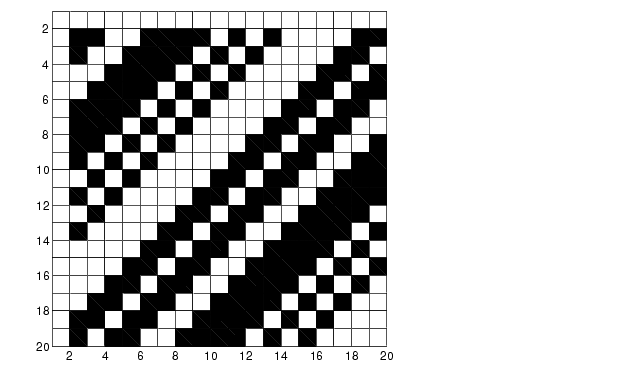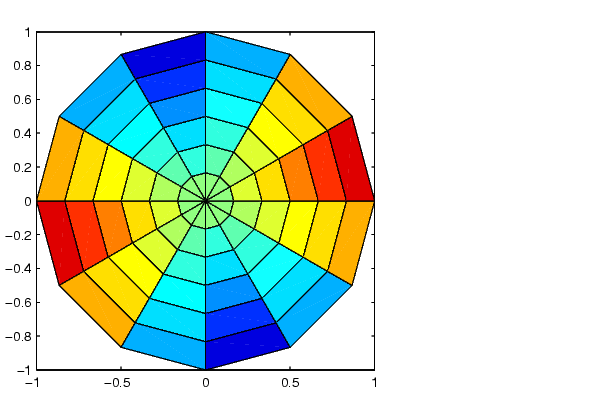MATLAB Function Referencepcolor

Pseudocolor plot

Syntax

• ```pcolor(C)
pcolor(X,Y,C)
h = pcolor(...)
```

Description

A pseudocolor plot is a rectangular array of cells with colors determined by `C`. MATLAB creates a pseudocolor plot by using each set of four adjacent points in `C` to define a surface patch (i.e., cell).

```pcolor(C) ``` draws a pseudocolor plot. The elements of `C` are linearly mapped to an index into the current colormap. The mapping from `C` to the current colormap is defined by `colormap` and `caxis`.

```pcolor(X,Y,C) ``` draws a pseudocolor plot of the elements of `C` at the locations specified by `X` and `Y`. The plot is a logically rectangular, two-dimensional grid with vertices at the points `[X(i,j),` `Y(i,j)]`. `X` and `Y` are vectors or matrices that specify the spacing of the grid lines. If `X` and `Y` are vectors, `X` corresponds to the columns of `C` and `Y` corresponds to the rows. If `X` and `Y` are matrices, they must be the same size as `C`.

```h = pcolor(...) ``` returns a handle to a surface graphics object.

Remarks

A pseudocolor plot is a flat surface plot viewed from above. `pcolor(X,Y,C)` is the same as viewing `surf``(X,Y,0*Z,C)` using `view``([0` `90])`.

When you use `shading` `faceted` or `shading` `flat`, the constant color of each cell is the color associated with the corner having the smallest x-y coordinates. Therefore, `C(i,j)` determines the color of the cell in the ith row and jth column. The last row and column of `C` are not used.

When you use `shading interp`, each cell's color results from a bilinear interpolation of the colors at its four vertices and all elements of `C` are used.

Examples

A Hadamard matrix has elements that are `+1` and `-1`. A colormap with only two entries is appropriate when displaying a pseudocolor plot of this matrix.

• ```pcolor(hadamard(20))
colormap(gray(2))
axis ij
axis square```

A simple color wheel illustrates a polar coordinate system.

• ```n = 6;
r = (0:n)'/n;
theta = pi`*`(-n:n)/n;
X = r`*`cos(theta);
Y = r`*`sin(theta);
C = r`*`cos(2*theta);
pcolor(X,Y,C)
axis equal tight```

Algorithm

The number of vertex colors for `pcolor(C)` is the same as the number of cells for `image(C)`.` pcolor` differs from `image` in that `pcolor(C)` specifies the colors of vertices, which are scaled to fit the colormap; changing the axes `clim` property changes this color mapping. `image(C)` specifies the colors of cells and directly indexes into the colormap without scaling. Additionally, `pcolor(X,Y,C)` can produce parametric grids, which is not possible with `image`.

`caxis`, `image`, `mesh`, `shading`, `surf`, `view`pcode pdepe